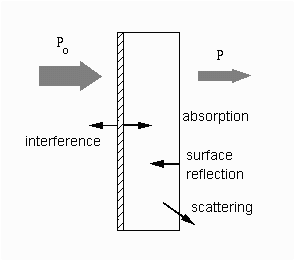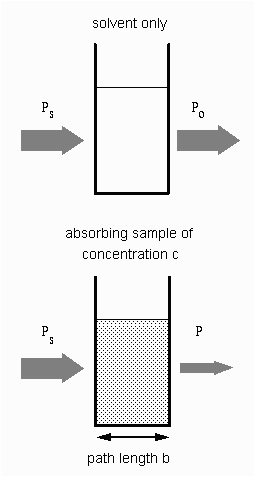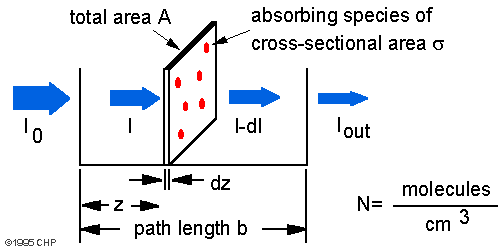# Beer-Lambert Law

## Introduction

The Beer-Lambert law (also called the Beer-Lambert-Bouguer law or simply Beer's law) is the linear relationship between absorbance and concentration of an absorber of electromagnetic radiation. The general Beer-Lambert law is usually written as:

A  =  aλ · b · c

where A is the measured absorbance, aλ is a wavelength-dependent absorptivity coefficient, b is the path length, and c is the analyte concentration. When working in concentration units of molarity, the Beer-Lambert law is written as:

A  =  el · b · c

where el is the wavelength-dependent molar absorptivity coefficient with units of M-1 cm-1. The λ subscript is often dropped with the understanding that a value for ε is for a specific wavelength. If multiple species that absorb light at a given wavelength are present in a sample, the total absorbance at that wavelength is the sum due to all absorbers:

A  =  (ε1 · b · c1) + (e2 · b · c2) + ...

where the subscripts refer to the molar absorptivity and concentration of the different absorbing species that are present.

## Theory

Experimental measurements are usually made in terms of transmittance (T), which is defined as:

T  =  P/Po

where P is the power of light after it passes through the sample and Po is the initial light power. The relation between A and T is:

A  =  − log(T)  = − log(P/Po)

The figure shows the case of absorption of light through an optical filter and includes other processes that decreases the transmittance such as surface reflectance and scattering.In analytical applications we often want to measure the concentration of an analyte independent of the effects of reflection, solvent absorption, or other interferences. The figure to the right shows the two transmittance measurements that are necessary to use absorption to determine the concentration of an analyte in solution. The top diagram is for solvent only and the bottom is for an absorbing sample in the same solvent. In this example, Ps is the source light power that is incident on a sample, P is the measured light power after passing through the analyte, solvent, and sample holder, and Po is the measured light power after passing through only the solvent and sample holder. The measured transmittance in this case is attributed to only the analyte. Depending on the type of instrument, the reference measurement (top diagram) might be made simultaneously with the sample measurement (bottom diagram) or a reference measurement might be saved on computer to generate the full spectrum. Absorption of light by a sampleModern absorption instruments can usually display the data as either transmittance, %-transmittance, or absorbance. An unknown concentration of an analyte can be determined by measuring the amount of light that a sample absorbs and applying Beer's law. If the absorptivity coefficient is not known, the unknown concentration can be determined using a working curve of absorbance versus concentration derived from standards.

## Derivation of the Beer-Lambert law

The Beer-Lambert law can be derived from an approximation for the absorption coefficient for a molecule by approximating the molecule by an opaque disk whose cross-sectional area, σ, represents the effective area seen by a photon of frequency w. If the frequency of the light is far from resonance, the area is approximately 0, and if w is close to resonance the area is a maximum. Taking an infinitesimal slab, dz, of sample:Io is the intensity entering the sample at z = 0, Iz is the intensity entering the infinitesimal slab at z, dI is the intensity absorbed in the slab, and I is the intensity of light leaving the sample. Then, the total opaque area on the slab due to the absorbers is σ · N · A · dz. Then, the fraction of photons absorbed will be σ · N · A · dz / A so,

dI/Iz  =  - s · N · dz

Integrating this equation from z = 0 to z = b gives:

ln(I) − ln(I0)  = − σ · N · b

or

− ln(I/Io)  =  σ · N · b

Since
N (molecules/cm³) · (1 mole/6.023·1023 molecules) · 1000 cm³ / liter = c (moles/liter)
and
2.303 · log(x) = ln(x)
then
- log(I / Io) = σ · (6.023x1020 / 2.303) · c · b
or
- log(I / Io) = A = ε · b · c
where
ε = σ · (6.023x1020 / 2.303) = σ · 2.61x1020

Typical cross-sections and molar absorptivities are:
 σ (cm2) ε (M-1 cm-1) absorption - atoms 10-12 3x108 absorption - molecules 10-16 3x104 absorption - infrared 10-19 3x10 Raman scattering 10-29 3x10-9

## Limitations of the Beer-Lambert law

The linearity of the Beer-Lambert law is limited by chemical and instrumental factors. Causes of nonlinearity include:
• deviations in absorptivity coefficients at high concentrations (>0.01M) due to electrostatic interactions between molecules in close proximity
• scattering of light due to particulates in the sample
• fluoresecence or phosphorescence of the sample
• changes in refractive index at high analyte concentration
• shifts in chemical equilibria as a function of concentration
• non-monochromatic radiation, deviations can be minimized by using a relatively flat part of the absorption spectrum such as the maximum of an absorption band
• stray light

## Related Topics

Auf diesem Webangebot gilt die Datenschutzerklärung der TU Braunschweig mit Ausnahme der Abschnitte VI, VII und VIII.